Open in App
Not now

# Pass by reference vs value in Python

• Difficulty Level : Medium
• Last Updated : 13 Jul, 2022

Developers jumping into Python programming from other languages like C++ and Java are often confused by the process of passing arguments in Python. The object-centric data model and its treatment of assignment is the cause behind the confusion at the fundamental level.

In the article, we will be discussing the concept of how to pass a value by reference in Python and try to understand pass-by-reference examples in Python.

## Pass by value and pass by reference in Python

You might want to punch something after reading ahead, so brace yourself. Python’s argument passing model is neither “Pass by Value” nor “Pass by Reference” but it is “Pass by Object Reference”.

The paradigms of “Pass by value”, “Pass by Reference” and “Pass by object Reference” can be understood by exploring the below example functions. Look into the two functions defined below:

## Python3

 `def` `set_list(``list``):``    ``list` `=` `[``"A"``, ``"B"``, ``"C"``]``    ``return` `list` `def` `add(``list``):``    ``list``.append(``"D"``)``    ``return` `list` `my_list ``=` `[``"E"``]` `print``(set_list(my_list))` `print``(add(my_list))`

Output:

```['A', 'B', 'C']
['E', 'D']```

Now, let’s explore the above code,

## The variable is not the object

Here “a” is a variable that points to a list containing the element “X” and “Y”. But “a” itself is not the list. Consider “a” to be a bucket that contains the object “X” and “Y”.

` a = ["X", "Y"]`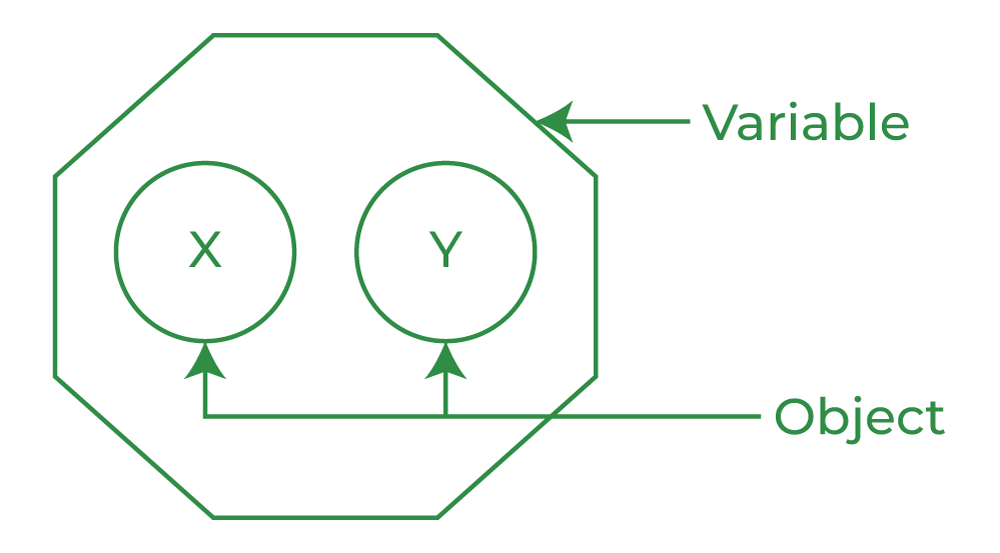## What is Pass by Reference In Python?

Pass by reference means that you have to pass the function(reference) to a variable which refers that the variable already exists in memory.

Here, the variable( the bucket) is passed into the function directly. The variable acts as a Package that comes with its contents(the objects).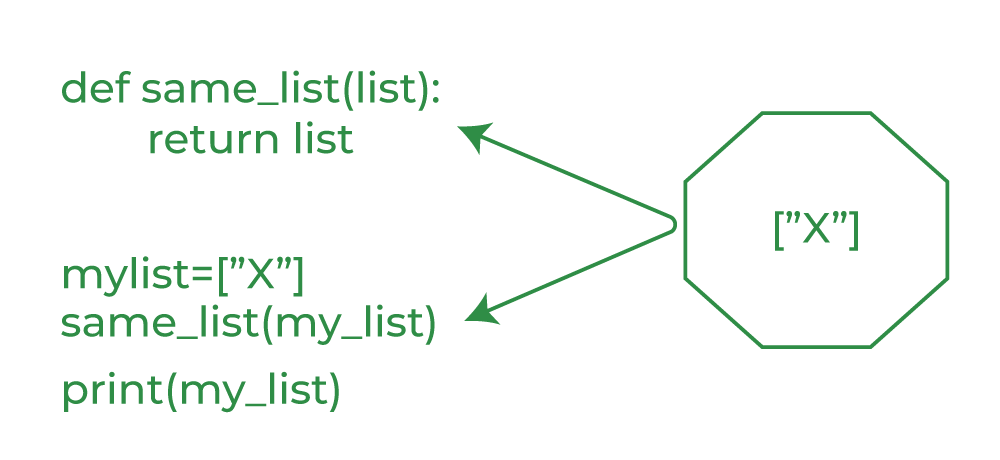In the above code image both “list” and “my_list” are the same container variable and therefore refer to the exact same object in the memory. Any operation performed by the function on the variable or the object will be directly reflected by the function caller. For instance, the function could completely change the variable’s content, and point it at a completely different object: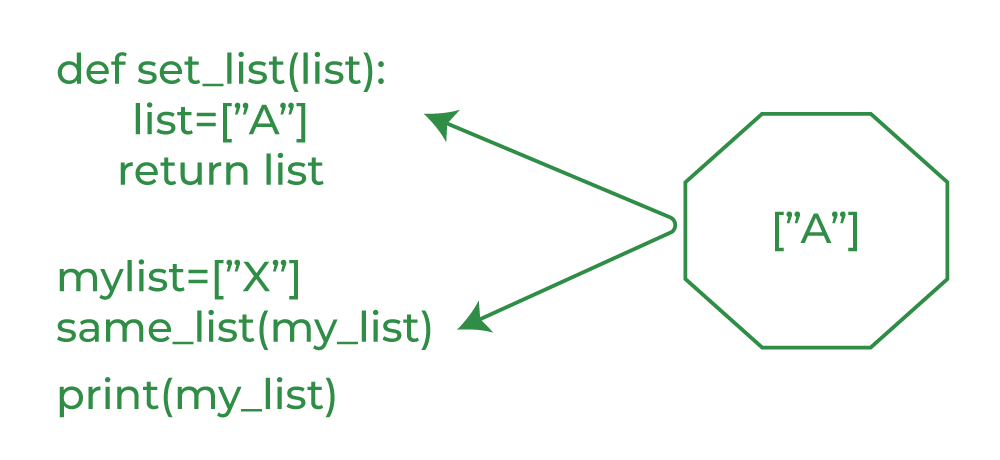Also, the function can reassign the contents of the variable with the same effect as below: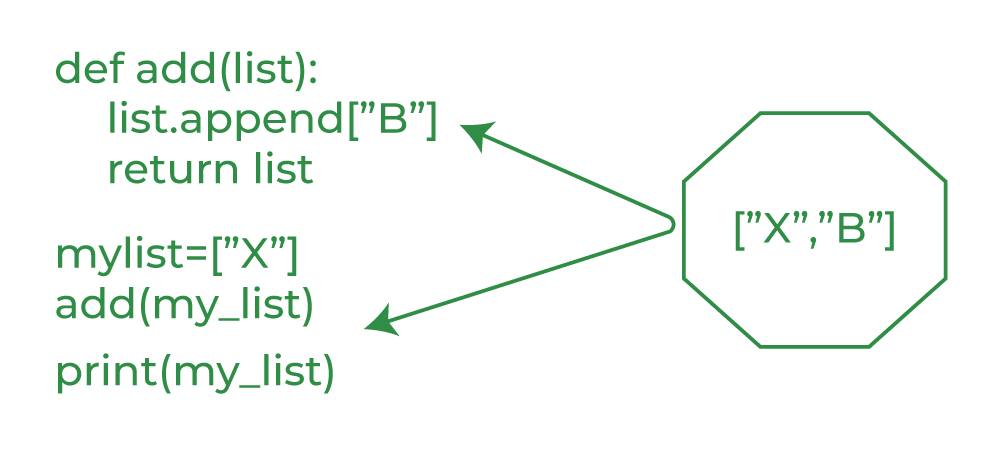To summarize, in pass-by-reference the function and the caller use the same variable and object.

## What is Pass by Value In Python?

In this approach, we pass a copy of actual variables in function as a parameter. Hence any modification on parameters inside the function will not reflect in the actual variable.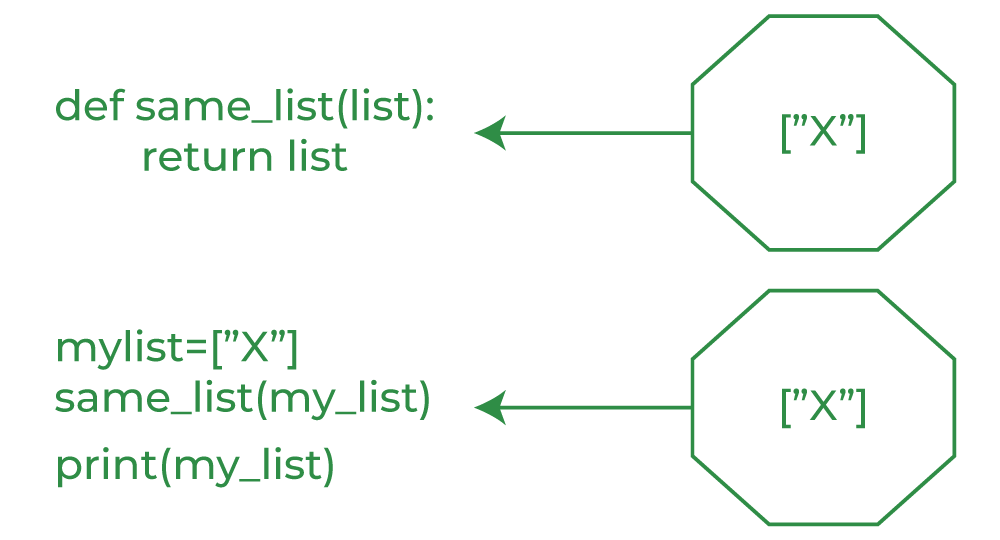The same is true for any operation performed by the function on the variable or the object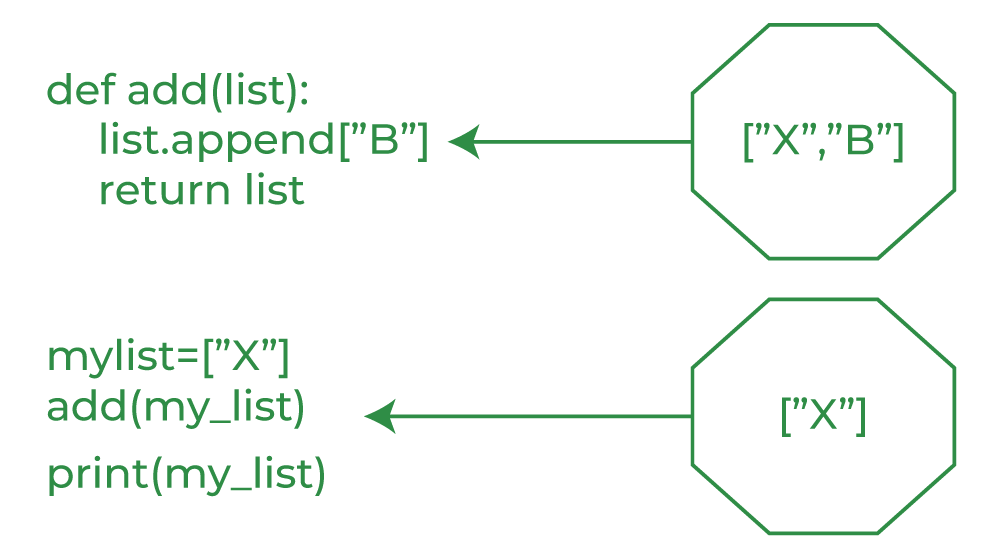To summarize the copies of the variables and the objects in the context of the caller of the function are completely isolated.

My Personal Notes arrow_drop_up